International
Tables for
Crystallography
Volume B
Reciprocal space
Edited by U. Shmueli

International Tables for Crystallography (2006). Vol. B. ch. 4.5, pp. 469-470   | 1 | 2 |

## Section 4.5.2.4.2. Polycrystalline fibres

R. P. Millanea*

#### 4.5.2.4.2. Polycrystalline fibres

| top | pdf |

A polycrystalline fibre is made up of crystallites that are oriented parallel to each other, but are randomly positioned and randomly rotated about their molecular axes. The recorded diffraction pattern is the intensity diffracted by a single crystallite, cylindrically averaged about the Z axis. On a fibre diffraction pattern, therefore, the Bragg reflections are cylindrically projected onto the (R, Z) plane and their positions are described by the cylindrically projected reciprocal lattice (Finkenstadt & Millane, 1998).

The molecules are periodic and are therefore usually aligned with one of the unit-cell vectors. Since the z axis is defined as the fibre axis, it is usual in fibre diffraction to take the c lattice vector as the unique axis and as the lattice vector parallel to the molecular axes. It is almost always the case that the fibre is rotationally disordered about the molecular axes, i.e. about c. Consider first the case of a monoclinic unit cell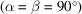so that the reciprocal lattice is cylindrically projected about. The cylindrical coordinates of the projected reciprocal-lattice points are then given by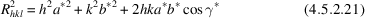and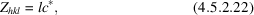so that R depends only on h and k, and Z depends only on l. Reflections with fixed h and k lie on straight row lines. Certain sets of distinct reciprocal-lattice points will have the same value of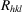and therefore superimpose in cylindrical projection. For example, for an orthorhombic system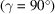the reciprocal-lattice points (hkl),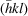,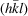and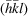superimpose. Furthermore, the crystallites in a fibre specimen are usually oriented randomly up and down so that the reciprocal-lattice points (hkl) and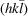superimpose, so that in the orthorhombic case eight reciprocal-lattice points superimpose. Also, as described below, reciprocal-lattice points that have similar values of R can effectively superimpose.

If the unit cell is either triclinic, or is monoclinic with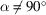or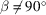, thenis inclined to c and the Z axis, and the reciprocal lattice is not cylindrically projected about. Equation (4.5.2.22)forstill applies, but the cylindrical radius is given by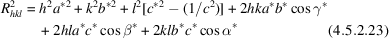and the row lines are curved (Finkenstadt & Millane, 1998).

The most complicated situation arises if the crystallites are rotationally disordered about an axis that is inclined to c. Reciprocal space is then rotated about an axis that is inclined to the normal to theplane,andare both functions of h, k and l, equation (4.5.2.23)does not apply, and reciprocal-lattice points for fixed l do not lie on layer lines of constant Z. Although this situation is rather unusual, it does occur (Daubeny et al., 1954), and is described in detail by Finkenstadt & Millane (1998).

The observed fibre diffraction pattern consists of reflections at the projected reciprocal-lattice points whose intensities are equal to the sums of the intensities of the contributing structure factors. The observed intensity, denoted by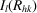, at a projected reciprocal-lattice point on the lth layer line and with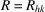is therefore given by (assuming, for simplicity, a monoclinic system)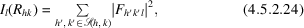where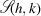denotes the set of indices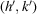such that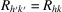. The number of independent reflections contributing in equation (4.5.2.24)depends on the space-group symmetry of the crystallites, because of either systematic absences or structure factors whose values are related.

The effect of a finite crystallite size is to smear what would otherwise be infinitely sharp reflections into broadened reflections of a finite size. If the average crystallite dimensions normal and parallel to the z axis are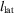(i.e. in the lateral' direction) and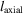(i.e. in the axial' direction), respectively, the profile of the reflection centred at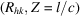can be written as (Fraser et al., 1984; Millane & Arnott, 1986; Millane, 1989c)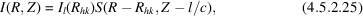where the profile function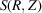can be approximated by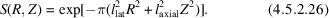The effect of crystallite disorientation is to smear the reflections given by equation (4.5.2.26)about the origin of the projected reciprocal space. If the effects of disorientation dominate over those of crystallite size, then the profile of a reflection can be approximated by (Fraser et al., 1984; Millane & Arnott, 1986; Millane, 1989c)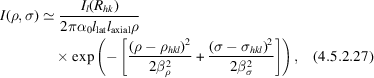where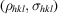are the polar coordinates of the reflection,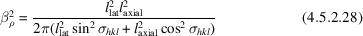and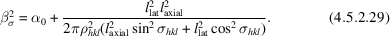Reflections that have similar enough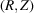coordinates overlap severely with each other and are also included in the sum in equation (4.5.2.24). This is quite common in practice because a number of sets of reflections may have similar values of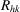.

### ReferencesDaubeny, R. de P., Bunn, C. W. & Brown, C. J. (1954). The crystal structure of polyethylene terephthalate. Proc. R. Soc. London Ser. A, 226, 531–542.Google ScholarFinkenstadt, V. L. & Millane, R. P. (1998). Fiber diffraction patterns for general unit cells: the cylindrically projected reciprocal lattice. Acta Cryst. A54, 240–248.Google ScholarFraser, R. D. B., Suzuki, E. & MacRae, T. P. (1984). Computer analysis of X-ray diffraction patterns. In Structure of crystalline polymers, edited by I. H. Hall, pp. 1–37. New York: Elsevier.Google ScholarMillane, R. P. (1989c). Relating reflection boundaries in X-ray fibre diffraction patterns to specimen morphology and their use for intensity measurement. J. Macromol. Sci. Phys. B28, 149–166.Google ScholarMillane, R. P. & Arnott, S. (1986). Digital processing of X-ray diffraction patterns from oriented fibres. J. Macromol. Sci. Phys. B24, 193–227.Google Scholar